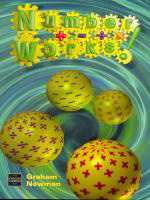Number Works!

By Graham Newman
December 2000
Nelson Thornes
ISBN: 0748754482250 pages, illustrated
\$26.50 paper original

Contents include: Number, addition, subtraction, times tables, multiplication, division, decimals, number properties and sequences, fractions (addition and subtraction), fractions (multiplication and division), ratio and proportion, percentages, measurement and tables, graphs and charts.